Name:    MCAS Conservation Laws - Momentum 2004-2014

Multiple Choice
Identify the choice that best completes the statement or answers the question.

1.

Which of the following has the least momentum? (MCAS 2006)
 a. a 0.5 kg mass with a 1000 m/s velocity c. a 10 kg mass with an 11 m/s velocity b. a 1 kg mass with a 100 m/s velocity d. a 100 kg mass with a 2 m/s velocity

2.

A pitcher throws a 0.15 kg baseball at 40 m/s towards the catcher. What is the momentum of the baseball while moving at 40 m/s? (MCAS 2006)
 a. 0.025 kg•m/s c. 6.0 kg•m/s b. 3.8 kg•m/s d. 270 kg•m/s

3.

A hockey player swings her hockey stick and strikes a puck. According to Newton’s third law of motion, which of the following is a reaction to the stick pushing on the puck? (MCAS 2007)
 a. the puck pushing on the stick c. the player pushing on the stick b. the stick pushing on the player d. the puck pushing on the player

4.

The diagrams below show a cart moving with a velocity, V, on a frictionless surface as a wooden block is being dropped. The block then falls straight down onto the moving cart. (MCAS 2007)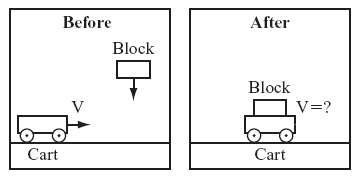Which of the following statements describes what will happen after the block lands on the moving cart?
 a. The cart will move to the left at a velocity less than the original velocity of the cart. b. The cart will move to the left at a velocity greater than the original velocity of the cart. c. The cart will move to the right at a velocity less than the original velocity of the cart. d. The cart will move to the right at a velocity greater than the original velocity of the cart.

5.

A 600 g basketball, a 57 g tennis ball, a 46 g golf ball, and a 2.7 g table tennis ball are moving with the same velocity. Which ball has the greatest momentum? (MCAS 2007)
 a. golf ball c. tennis ball b. basketball d. table tennis ball

6.

To calculate the momentum of a pickup truck with a velocity of 25 m/s east, it is also necessary to know which of the following? (MCAS 2007)
 a. time elapsed c. mass of the pickup truck b. drive force of the motor d. distance that the pickup travels

7.

Mike, who has a mass of 75 kg, is running north at 2.6 m/s. He accidentally collides with Tom, who has a mass of 125 kg and is not moving. Which of the following statements describes how much momentum each person has before the collision? (MCAS 2008)
 a. Mike has a momentum of 130 kg•m/s north, and Tom has no momentum. b. Mike has a momentum of 195 kg•m/s north, and Tom has no momentum. c. Both Mike and Tom have a momentum of 130 kg•m/s north. d. Both Mike and Tom have a momentum of 195 kg•m/s north.

8.

A cart with a mass of 5 kg rests on a floor next to a wall, as shown in the diagram below. (MCAS 2008)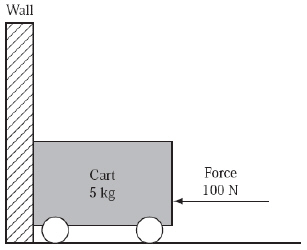A person pushes on the cart to the left toward the wall with a force of 100 N. Which of the following statements is true in this situation?
 a. The wall does not push on the cart. b. The floor pushes 100 N up on the cart. c. The cart pushes 100 N down on the floor. d. The wall pushes 100 N to the right on the cart.

9.

What is the momentum of a metal disc with a mass of 1.5 kg sliding on a frictionless surface at 0.75 m/s? (MCAS 2008)
 a. 0.50 kg•m/s c. 1.1 kg•m/s b. 0.85 kg•m/s d. 2.0 kg•m/s

10.

A 1 kg block sliding to the right on a level, frictionless surface with a speed of 2 m/s collides and sticks to a second 1 kg block sliding to the left.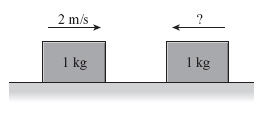After the collision, the blocks are motionless. What was the speed of the second 1 kg block before the collision? (MCAS 2009)
 a. 1 m/s c. 3 m/s b. 2 m/s d. 4 m/s

11.

Which of the following has the greatest momentum? (MCAS 2009)
 a. a 60 kg deer moving west at a speed of 16.4 m/s b. a 6300 kg elephant moving east at a speed of 0.11 m/s c. a 0.42 kg soccer ball moving downfield at a speed of 12 m/s d. a 0.03 kg arrow moving toward a target at a speed of 150 m/s

12.

A student is standing on a skateboard that is not moving. The total mass of the student and the skateboard is 50 kilograms. The student throws a ball with a mass of 2 kilograms forward at 5 m/s. Assuming the skateboard wheels are frictionless, how will the student and the skateboard move? (MCAS 2005)
 a. forward at 0.4 m/s c. backward at 0.2 m/s b. forward at 5 m/s d. backward at 5 m/s

13.

What is the mass of an asteroid with a speed of 200 m/s and a momentum of 2,000 kgm/s? (MCAS 2004)
 a. 10 kg c. 2,200 kg b. 1,800 kg d. 400,000 kg

14.

A bowling ball with a mass of 8.0 kg rolls down a bowling lane at 2.0 m/s. What is the momentum of the bowling ball? (MCAS 2004)
 a. 4 c. 10 b. 6 d. 16

15.

The momentum of an object in space is (MCAS 2010)
 a. dependent on its mass. c. independent of its velocity. b. independent of its inertia. d. dependent on its potential energy.

16.

At an amusement park, bumper car X moves at a speed of 2.5 m/s toward car Y, which is at rest, as shown in the diagram below.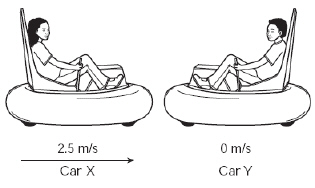Car X collides with car Y. How does the momentum of each car change after the collision? (MCAS 2010)
 a. Car X’s momentum increases, and car Y’s momentum decreases. b. Car X’s momentum decreases, and car Y’s momentum increases. c. Car X’s momentum is unchanged, and car Y’s momentum increases. d. Car X’s momentum is unchanged, and car Y’s momentum decreases.

17.

Two students are standing next to each other on a level field. One of the students throws a table tennis ball forward toward a line 2.0 m away. At the same time, the second student throws a bowling ball in the same direction. Both balls take the same time to travel the 2.0 m. Only the second student feels a noticeable backward push when she throws her ball. Which of the following statements explains why only the second student feels a noticeable backward push? (MCAS 2010)
 a. The bowling ball exerts a much larger reaction force. b. The bowling ball undergoes a much larger acceleration. c. The bowling ball requires more force to overcome gravity. d. The bowling ball converts more inertia into kinetic energy.

18.

A person with a weight of 120 lb. is standing on a scale.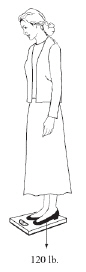What force is the scale exerting on the person? (MCAS 2011)
 a. 0 lb. up c. 120 lb. up b. 60 lb. up d. 240 lb. up

19.

A student is driving her car when an insect strikes her windshield. Which of the following statements best describes the forces in this situation? (MCAS 2011)
 a. The insect strikes the windshield with the same force as the windshield strikes the insect. b. The insect strikes the windshield with a force, and the windshield exerts no force on the insect. c. The insect exerts no force on the windshield, and the windshield strikes the insect with a large force. d. The insect strikes the windshield with a small force, and the windshield strikes the insect with a large force.

20.

Two toy carts are about to collide,as shown in the diagram below.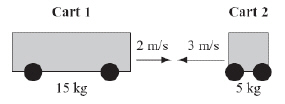Cart 1 has a mass of 15 kg and is moving right at a speed of 2 m/s. Cart 2 has a mass of 5 kg and is moving left at a speed of 3 m/s. When they collide, the carts will stick together.
Neglecting friction, what will be the velocity of the carts after the collision? (MCAS 2011)
 a. 0.75 m/s left c. 15 m/s left b. 0.75 m/s right d. 15 m/s right

21.

A meteoroid with a mass of 1,000 kg enters Earth’s atmosphere with a velocity of 4,400 m/s. What is the magnitude of the meteoroid’s momentum at that instant? (MCAS 2011)
 a. 44 kg·m/s c. 4,400,000 kg·m/s b. 2,200,000 kg·m/s d. 9,680,000,000 kg·m/s

22.

An object with a mass of 3 kg has a momentum of 75 kgm/s. What is its velocity? (MCAS 2012)
 a. 0.4 m/s c. 25 m/s b. 7.1 m/s d. 72 m/s

23.

A 20 kg child is traveling 3 m/s on an amusement park ride. What is the magnitude of the child’s momentum? (MCAS 2012)
 a. 6.7 kg • m/s c. 90 kg • m/s b. 60 kg • m/s d. 200 kg • m/s

24.

What is the magnitude of the momentum of a 0.50 kg ball moving in a straight line at 5.0 m/s? (MCAS 2013)
 a. 0.1 kg • m/s c. 6.3 kg • m/s b. 2.5 kg • m/s d. 10 kg • m/s

25.

Which of the following has the greatest momentum? (MCAs 2013)
 a. 0.2 kg ball moving at 40 m/s c. 2000 kg truck traveling at 9 m/s b. 500 kg car traveling at 16 m/s d. 50 kg child skateboarding at 4 m/s

26.

A worker in a warehouse pushes two wooden boxes across a floor at a constant speed, as shown in the diagram below.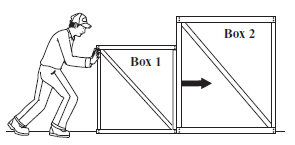The arrow in the diagram represents the force box 1 exerts on box 2. Which arrow represents the reaction force?  (MCAS 2013)
 a.c.b.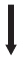d.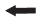27.

What is the magnitude of the momentum of a 0.15 kg baseball moving at 25 m/s? (MCAS 2014)
 a. 3.8 kg • m/s c. 167 kg • m/s b. 47 kg • m/s d. 1110 kg • m/s

Essay

28.

The illustrations below show an air track with two carts before and after a collision. The mass and the initial velocity of each cart are shown below. (MCAS 2006)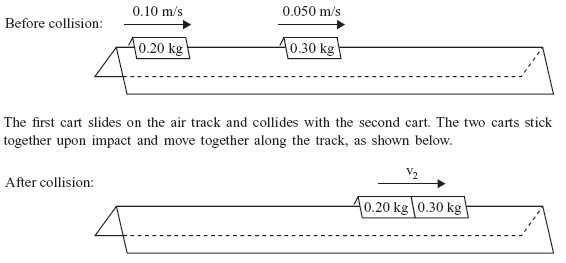a.       What is the momentum of the first cart before it collides with the second cart? Show your calculations and include units in your answer.

b.       What is the momentum of the second cart before the collision? Show your calculations and include units in your answer.

c.       Describe two changes that could be made initially to either one or both carts that would result in an increase in the momentum of the combined carts after the collision.

d.       What is the final velocity of the combined carts after the collision? Show your calculations and include units in your answer.

29.

On a smooth, level surface, a red marble of mass 0.02 kg moving at 2.0 m/s collides with a stationary yellow marble of equal mass. After the collision, the red marble stops completely, and the yellow marble moves in the direction the red marble was moving. (MCAS 2007)

a. Calculate the momentum of both marbles before the collision. Show your calculations and include units in your answer.

b. Calculate the momentum of both marbles after the collision. Show your calculations and include units in your answer.

c. If the velocity of the red marble doubles, how will the velocity of the yellow marble change after the collision?

d. If the red marble had more mass than the yellow marble, how would the momentum of the yellow marble change after the collision?